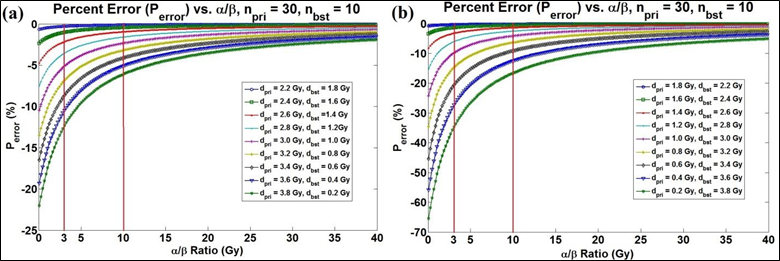Mathematical analysis of approximate biological effective dose (BED) calculation for multi-phase radiotherapy treatment plans

Mathematical analysis of approximate biological effective dose (BED) calculation for multi-phase radiotherapy treatment plans

Department of Radiation Oncology, University of Texas Health Science Center at San Antonio, San Antonio, TX, USA.
Cancer Therapy and Research Center, San Antonio, TX, USA.

Cite this article as: Kauweloa KI, Gutierrez AN, Bergamo A, Stathakis S, Papaniko-laou N, Mavroidis P. Mathematical analysis of approximate biological effective dose (BED) calculation for multi-phase radiotherapy treatment plans. Int J Cancer Ther Oncol 2014; 2(2):020226.
DOI:
http://dx.doi.org/10.14319/ijcto.0202.26

Conference Proceeding
[Presented at the Young Investigator’s Symposium at the 2014 Annual Meeting of Southwest Chapter of American Association of Physicists in Medicine (AAPM) in San Antonio, Texas, USA]

Abstract

Purpose: There is growing interest about biological effective dose (BED) and its application in treatment plan evaluation due to its stronger correlation with treatment outcome. An approximate biological effective dose (BEDA) equation was introduced in order to simplify BED calculations by treatment planning systems in multi-phase treatments. The purpose of this work is to reveal its mathematical properties relative to the true, multi-phase BED (BEDT) equation.

Methods: The BEDT equation was derived and used to reveal the mathematical properties of BEDA. MATLAB (MathWorks, Natick, MA) was used to simulate and analyze common and extreme clinical multi-phase cases. In those cases, percent error and Bland-Altman analysis were used to study the significance of the inaccuracies of BEDA for different combinations of total doses, numbers of fractions, doses per fractions and α/β values. All the calculations were performed on a voxel-basis in order to study how dose distributions would affect the accuracy of BEDA.

Results: When the voxel dose-per-fractions (DPF) delivered by both phases are equal, BEDA and BEDT are equal (0% error). In heterogeneous dose distributions, which significantly vary between the phases, there are fewer occurrences of equal DPFs and hence the imprecision of BEDA is greater. It was shown that as the α/β ratio increased the accuracy of BEDA would improve. Examining twenty-four cases, it was shown that the range of DPF ratios for 3% Perror varied from 0.32 to 7.50Gy, whereas for Perror of 1% the range varied from 0.50 to 2.96Gy.

Conclusion: The DPF between the different phases should be equal in order to render BEDA accurate. OARs typically receive heterogeneous dose distributions hence the probability of equal DPFs is low. Consequently, the BEDA equation should only be used for targets or OARs that receive uniform or very similar dose distributions by the different treatment phases.

Impact: To our knowledge, it is a usual practice to use BEDA instead of BEDT due to its convenience with current treatment planning systems (TPS). This was shown to be a potential problem with our mathematical analysis of BEDA. The goal of this work was to quantitatively measure the accuracy of BEDA under many different circumstances, and also reveal its potential imprecision in clinical circumstances regarding healthy organs. This work puts emphasis on the importance of creating future TPS with the capability of calculating the BEDT.

Key Results: Using the multi-phase equations of BEDT and BEDA, specifically two-phases (e.g., primary and boost), the percent error (Perror) equation was derived. Five variables within this equation are the α/β ratio, which is a tissue-dependent constant of the linear-quadratic (LQ) cell- survival curve model, the dose-per-fractions (DPF) for both phases, and the number of fractions for both phases. The Perror equation reveals the many varying factors in the accuracy of BEDA. Figure 1 displays the change of Perror with respect to four of the variables, except α/β, which is instead shown in Figure 2. As seen, the main determining factor in the accuracy of BEDA is the DPF-ratio, which, when it gets equal to 1, Perror becomes equal to 0% (see Figure 1). Since DPF- ratio is the most important factor in the accuracy of BEDA, and due to its dependence upon the dose distribution, we have shown that using BEDA on OARs could potentially lead to significant errors. On the other hand, its application to target volumes would instead produce less error due to fact that target volumes typically receive more homogeneous dose distributions.FIG. 1: As seen in all three plots, as the DPF-ratio steers from 1, Perror increases. Also, as npri/nbst increases, Perror decreases. The DPF-ratiomax and DPF-ratiomin reveal the ratios that lead to a 3% error of BEDA.FIG. 2: (a) This is a plot with DPF-ratios > 1, while (b) is with DPF-ratios < 1. When dpri/dbst is less than one, notice the steeper drop in Perror with a change in DPF.

Received March 19, 2014; Published Online April 08, 2014

Presenting author: Kevin Kauweloa; Department of Radiation Oncology, University of Texas Health Science Center at San Antonio, San Antonio, TX, USA.

#### Comments on this article

View all commentsThis work is licensed under a Creative Commons Attribution 3.0 License.

------------------------------------------------------------

International Journal of Cancer Therapy and Oncology (ISSN 2330-4049)

© International Journal of Cancer Therapy and Oncology (IJCTO)

To make sure that you can receive messages from us, please add the 'ijcto.org' domain to your e-mail 'safe list'. If you do not receive e-mail in your 'inbox', check your 'bulk mail' or 'junk mail' folders.

------------------------------------------------------------

Number of visits since October, 2013